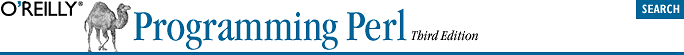home | O'Reilly's CD bookshelfs | FreeBSD | Linux | Cisco | Cisco Exam## 31.11. use integer

```use integer;
\$x = 10/3;
# \$x is now 3, not 3.33333333333333333```

This lexically scoped pragma tells the compiler to use integer operations from here through the end of the enclosing block. On many machines, this doesn't matter a great deal for most computations, but on those few remaining architectures without floating-point hardware, it can amount to a dramatic performance difference.

Note that this pragma affects certain numeric operations, not the numbers themselves. For example, if you run this code:

```use integer;
\$x = 1.8;
\$y = \$x + 1;
\$z = -1.8;```
you'll be left with \$x == 1.8, \$y == 2 and \$z == -1. The \$z case happens because unary - counts as an operation, so the value 1.8 is truncated to 1 before its sign bit is flipped. Likewise, functions that expect floating-point numbers, such as sqrt or the trig functions, still receive and return floats even under use integer. So sqrt(1.44) is 1.2, but 0 + sqrt(1.44) is now just 1.

Native integer arithmetic as provided by your C compiler is used. This means that Perl's own semantics for arithmetic operations might not be preserved. One common source of trouble is the modulus of negative numbers. Perl may do it one way, but your hardware may do it another:

```% perl -le 'print (4 % -3)'
-2
% perl -Minteger -le 'print (4 % -3)'
1```